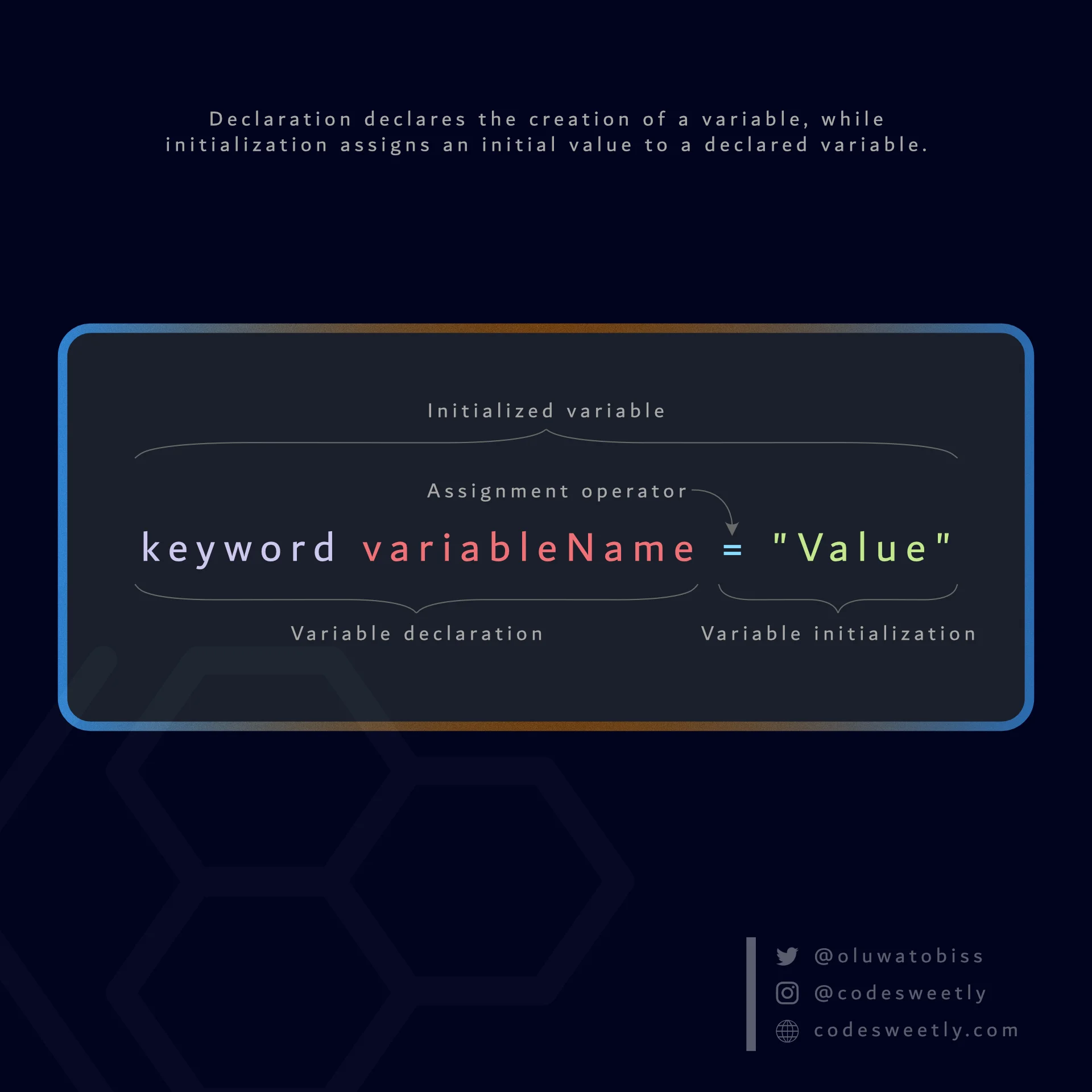# Declaration vs Initialization vs Invocation in Programming

Declaration, initialization, and invocation are three popular programming terms. But what exactly do they mean? Let's find out.

## What Exactly Does Declaration Mean?​

Declaration means to declare the creation of variables and functions.

Here's an example:

``var color;``

The code above is a declaration of a JavaScript `var` variable named `color`.

Notice that we did not store anything in the `color` variable.

In other words, a declaration is not concerned with the storage (initialization) of values. Instead, its job is to declare the creation of variables and functions.

Here's another example:

``function multiplyNumbers() {}``

The statement above declares that we've created a JavaScript function named `multiplyNumbers`.

## What Does Initialization Mean?​

Initialization occurs when you assign an initial value to a variable.

Here's a visual representation:Here's an example:

``const color = "green";``

In the snippet above, we initialized the `color` variable with an initial value of `"green"`.

Here's another example:

``let multiplyNumbers = function () {};``

In the snippet above, we initialized the `multiplyNumbers` variable with an initial value of a function.

Keep in mind that when the computer reads an initialized variable, it first evaluates the expression on the right of the assignment operator. Then, it assigns the resolved value to the variable on the left side.

For instance, in the snippet below, the computer will first evaluate `70 + 90`. Then, after the evaluation, it will assign the resolved value (`160`) to the `finalAnswer` variable.

``const finalAnswer = 70 + 90;``

## What Does Invocation Mean in Programming?​

Invocation means to execute a piece of code.

Here's an example:

``var color = "green";// Invoke the color variable:color;// The invocation above will return:"green"``

Here's another example:

``function multiplyNumbers(a, b) {  return a * b;}// Invoke the multiplyNumbers function:multiplyNumbers(3, 100);// The invocation above will return:300``

## In Summary​

• Declaration declares the creation of variables and functions.
• Initialization assigns initial values to variables.
• Invocation executes a piece of code.